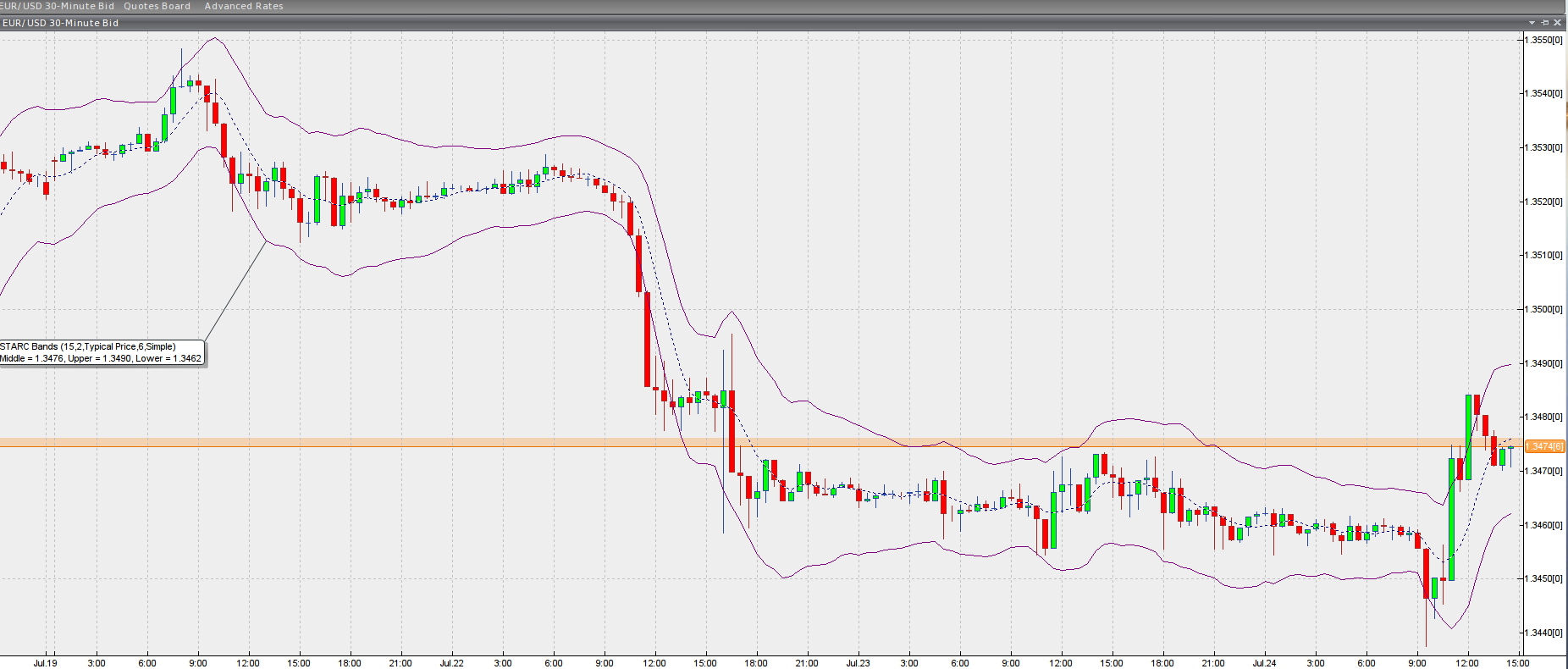# STARC Bands

## Stoller Average Range Channels

### This lesson will cover the following

• Definition
• Calculation
• Interpretation

Developed by Manning Stoller, the Stoller Average Range Channels are an indicator designed to encompass most of the price action, just like every other banding indicator, and thus pinpoint higher-probability entry points when the price crosses through any of its boundaries. The channel expands and contracts in accordance to changes in the Average True Range, the indicators measure of volatility.

The so-called STARC Bands are plotted around a simple moving average of the underlying assets price, thus the SMA is its core. Each boundarys position is then calculated by adding or subtracting the value of the ATR to/from the SMA. Here are the formulas:

## Best Forex Brokers for United States

Upper STARC band = SMA + ATR
Lower STARC band = SMA – ATR

Keep in mind, however, that before the calculation is executed, ATR is multiplied by a user-specific multiplier factor, which is most often 2. Commonly, the Simple Moving Average tracks back six periods. Thus, generally the more comprehensive formula (dont forget the variables are situational) looks as follows:

Upper STARC band = 6-period SMA + ATR*2
Lower STARC band = 6-period SMA – ATR*2

Here is what the indicator looks like when plotted in a trading platform.